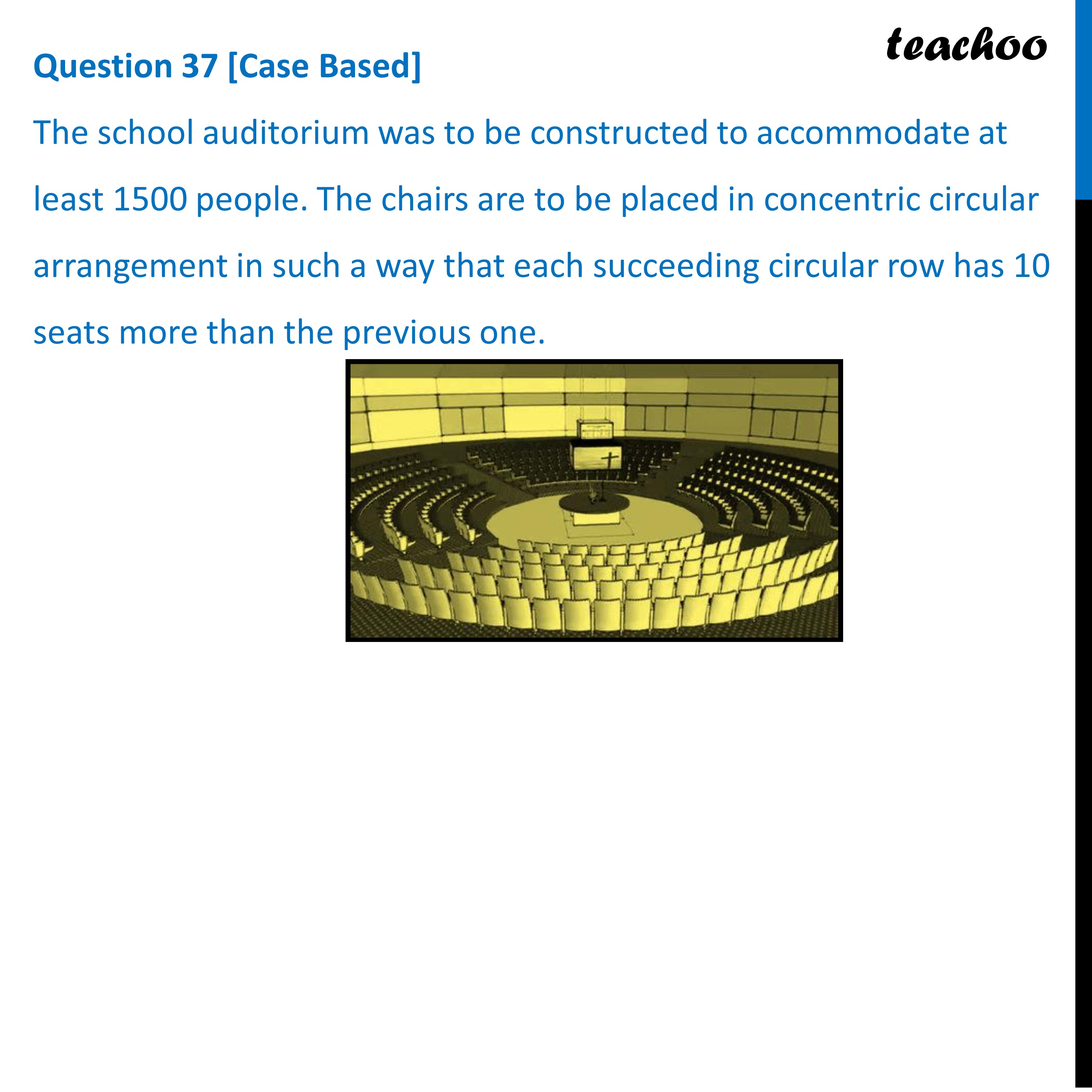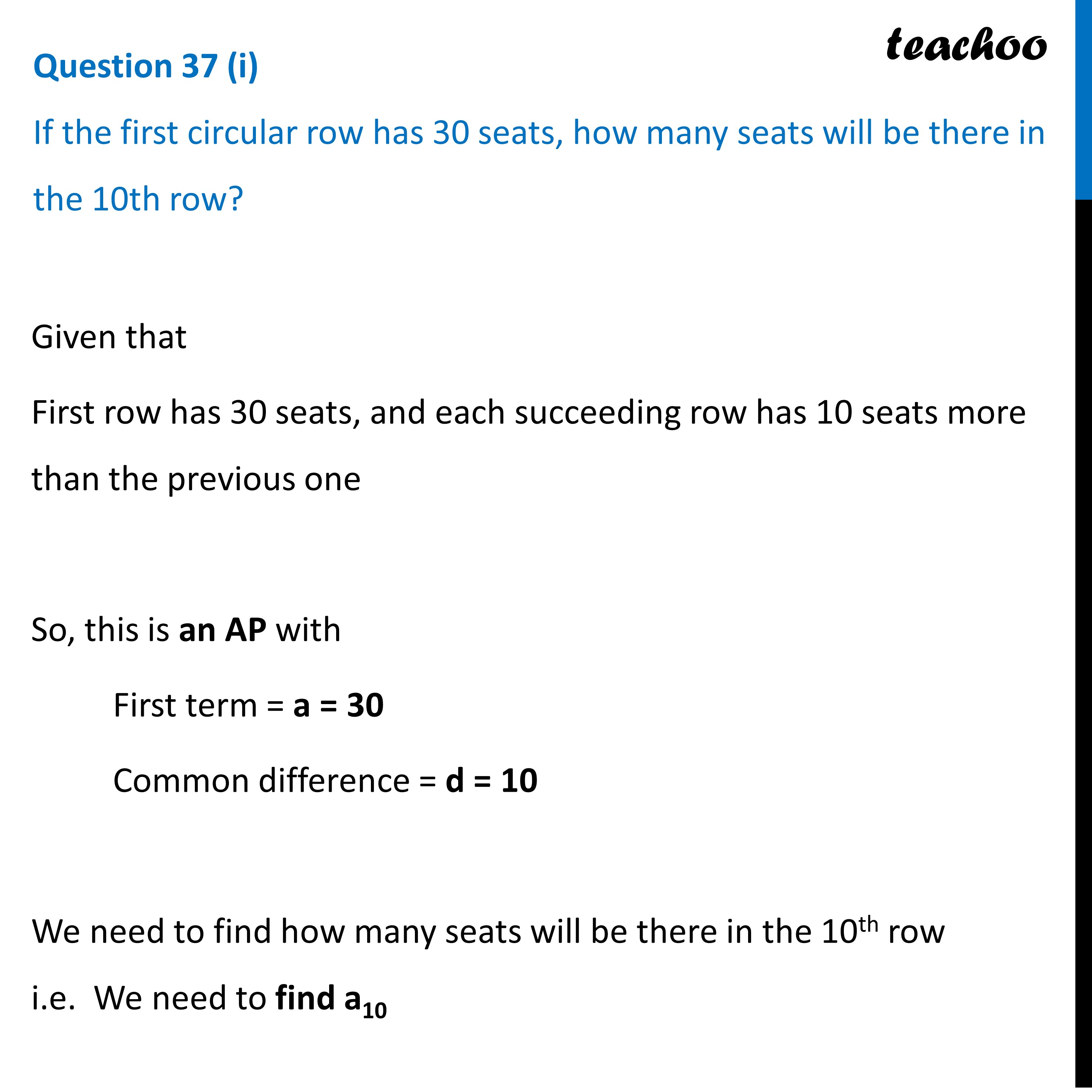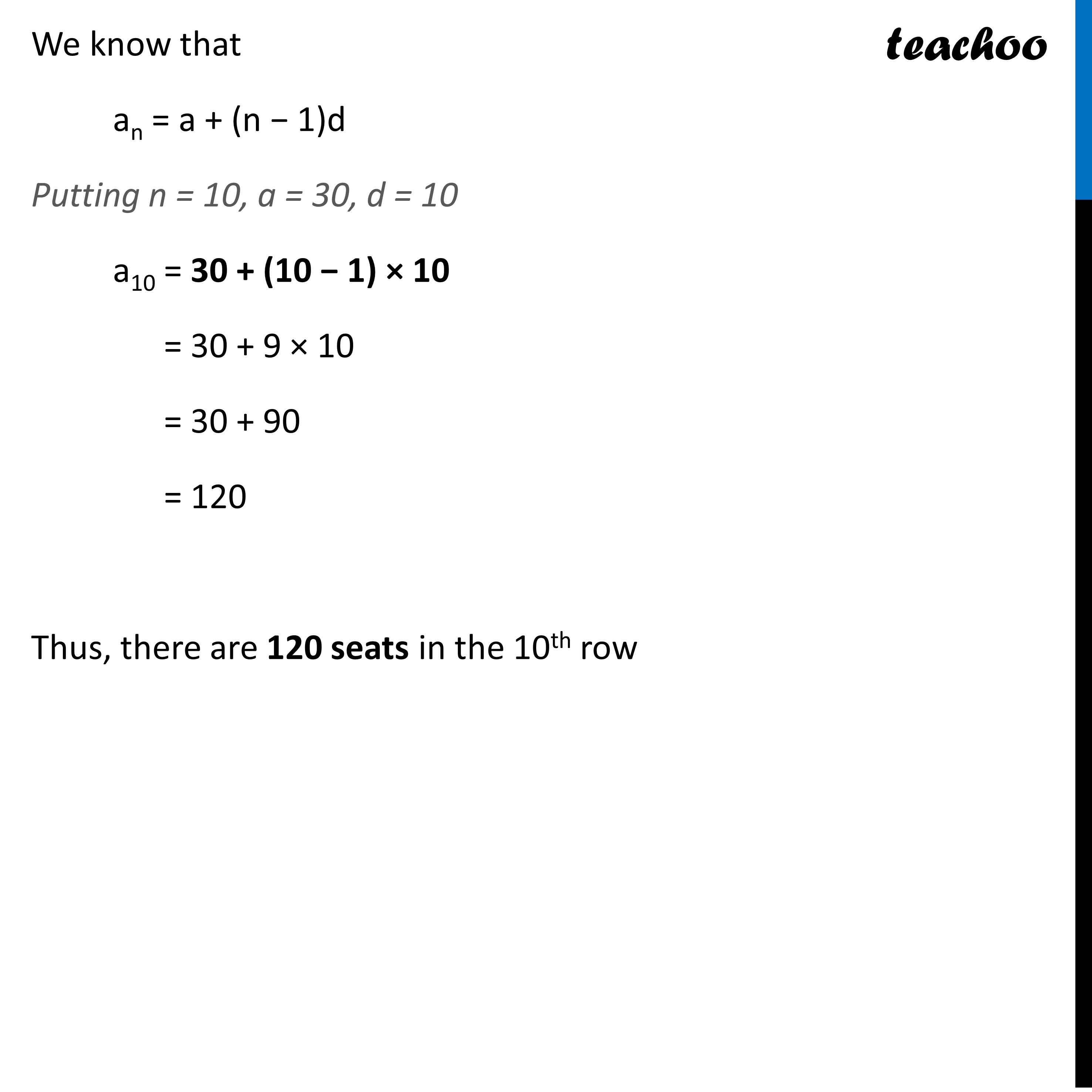CBSE Class 10 Sample Paper for 2023 Boards - Maths Standard

Class 10
Solutions of Sample Papers for Class 10 Boards

## If the first circular row has 30 seats, how many seats will be there in the 10th row?Learn in your speed, with individual attention - Teachoo Maths 1-on-1 Class

### Transcript

Question 37 [Case Based] The school auditorium was to be constructed to accommodate at least 1500 people. The chairs are to be placed in concentric circular arrangement in such a way that each succeeding circular row has 10 seats more than the previous one. Question 37 (i) If the first circular row has 30 seats, how many seats will be there in the 10th row? Given that First row has 30 seats, and each succeeding row has 10 seats more than the previous one So, this is an AP with First term = a = 30 Common difference = d = 10 We need to find how many seats will be there in the 10th row i.e. We need to find a10 We know that an = a + (n − 1)d Putting n = 10, a = 30, d = 1- a10 = 30 + (10 − 1) × 10 = 30 + 9 × 10 = 30 + 90 = 120 Thus, there are 120 seats in the 10th row